### 11.10 Examples

#### 11.10.1 Kovasznay Flow 2D

This example demonstrates the use of the velocity correction o solve the 2D Kovasznay flow at Reynolds number Re = 40. In the following we will numerically solve for the two dimensional velocity and pressure fields with steady boundary conditions.

##### 11.10.1.1 Input file

The input for this example is given in the example file KovaFlow_m8.xml. The mesh consists of 12 quadrilateral elements.

We will use a 7th-order polynomial expansions (N = 8 modes) using the modified Legendre basis and therefore require the following expansion definition.

1<EXPANSIONS>
2  <E COMPOSITE="C" NUMMODES="6" FIELDS="u,v,p" TYPE="MODIFIED" />
3</EXPANSIONS>

We next specify the time integration scheme and solver information for our problem. In particular, we select the velocity correction scheme formulation, using a continuous Galerkin projection. For this scheme, an implicit-explicit time-integration scheme must be used and we choose one of second order.

1 <TIMEINTEGRATIONSCHEME>
2    <METHOD> IMEX </METHOD>
3    <ORDER> 2 </ORDER>
4 </TIMEINTEGRATIONSCHEME>
5
6 <SOLVERINFO>
8    <I PROPERTY="SolverType"            VALUE="VelocityCorrectionScheme" />
10    <I PROPERTY="Projection"            VALUE="Galerkin"                 />
11 </SOLVERINFO>

The key parameters are listed below. Since the problem is unsteady we prescribe the time step and the total number of time steps. We also know the required Reynolds number, but we must prescribe the kinematic viscosity to the solver. We first define a dummy parameter for the Reynolds number, and then define the kinematic viscosity as the inverse of this. The value of λ is used when defining the boundary conditions and exact solution. Note that PI is a pre-defined constant.

1<PARAMETERS>
2  <P> TimeStep      = 0.001  </P>
3  <P> NumSteps      = 100    </P>
4  <P> Re            = 40     </P>
5  <P> Kinvis        = 1/Re   </P>
6  <P> LAMBDA        = 0.5*Re-sqrt(0.25*Re*Re+4*PI*PI)</P>
7</PARAMETERS>

We choose to impose a mixture of boundary condition types as defined below.

1<BOUNDARYCONDITIONS>
2    <REGION REF="0">
3        <D VAR="u" VALUE="1-exp(LAMBDA*x)*cos(2*PI*y)" />
4        <D VAR="v" VALUE="(LAMBDA/2/PI)*exp(LAMBDA*x)*sin(2*PI*y)" />
5        <N VAR="p" USERDEFINEDTYPE="H" VALUE="0" />
6    </REGION>
7    <REGION REF="1">
8        <D VAR="u" VALUE="1-exp(LAMBDA*x)*cos(2*PI*y)" />
9        <D VAR="v" VALUE="(LAMBDA/2/PI)*exp(LAMBDA*x)*sin(2*PI*y)" />
10        <D VAR="p" VALUE="0.5*(1-exp(2*LAMBDA*x))" />
11    </REGION>
12    <REGION REF="2">
13        <N VAR="u" VALUE="0" />
14        <D VAR="v" VALUE="0" />
15        <N VAR="p" VALUE="0" />
16    </REGION>
17</BOUNDARYCONDITIONS>

Initial conditions are obtained from the file KovaFlow_m8.rst, which is a Nektar++ field file. This is the output of an earlier simulation, renamed with the extension rst to avoid being overwritten, and is used in this case to reduce the integration time necessary to obtain the steady flow.

1<FUNCTION NAME="InitialConditions">
2    <F FILE="KovaFlow_m8.rst" />
3</FUNCTION>

Note the use of the F tag to indicate the use of a file. In contrast, the exact solution is prescribed using analytic expressions which requires the use of the E tag.

1<FUNCTION NAME="ExactSolution">
2    <E VAR="u" VALUE="1-exp(LAMBDA*x)*cos(2*PI*y)" />
3    <E VAR="v" VALUE="(LAMBDA/2/PI)*exp(LAMBDA*x)*sin(2*PI*y)" />
4    <E VAR="p" VALUE="0.5*(1-exp(2*LAMBDA*x))" />
5</FUNCTION>

##### 11.10.1.2 Running the simulation

Launch the simulation using the following command

IncNavierStokesSolver KovaFlow_m8.xml

After completing the prescribed 100 time-steps, the difference between the computed solution and the exact solution will be displayed. The actual mantissas may vary slightly, but the overall magnitude should be as shown.

L 2 error (variable u) : 3.75296e-07
L inf error (variable u) : 5.13518e-07
L 2 error (variable v) : 1.68897e-06
L inf error (variable v) : 2.23918e-06
L 2 error (variable p) : 1.46078e-05
L inf error (variable p) : 5.18682e-05

The output of the simulation is written to KovaFlow_m8.fld. This can be visualised by converting it to a visualisation format. For example, to use ParaView, convert the output into VTK format using the tility.

FieldConvert KovaFlow.xml KovaFlow.fld KovaFlow.vtu

The result should look similar to that shown in Figure 11.4.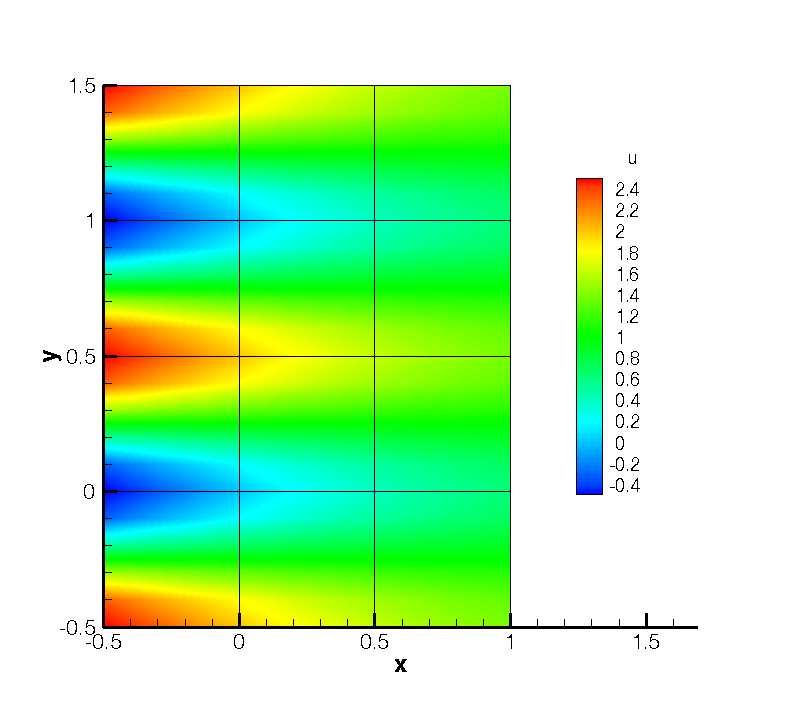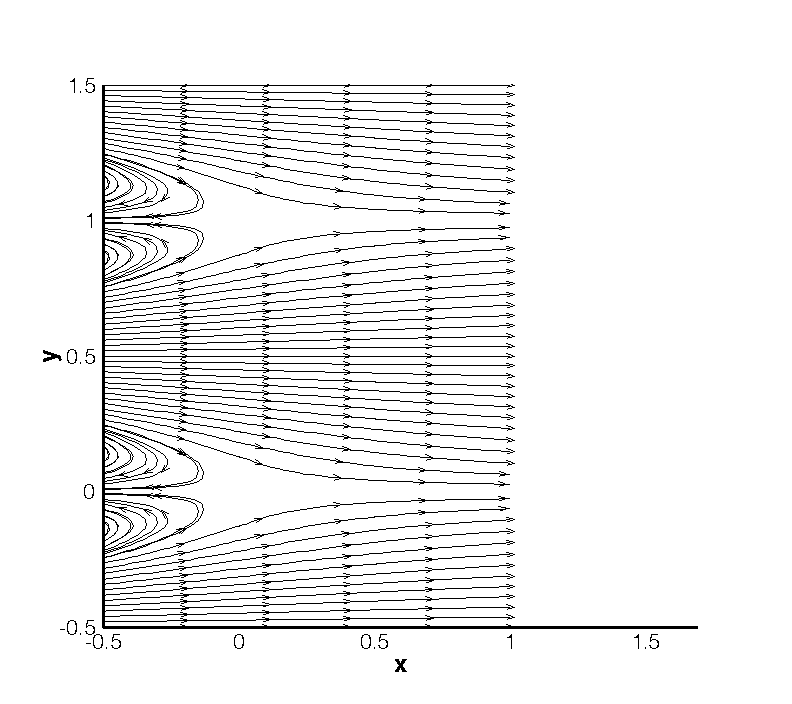Figure 11.4: Velocity profiles for the Kovasznay Flow (2D).

#### 11.10.2 Kovasznay Flow 2D using high-order outflow boundary conditions

In this example, we solve the same case of 2D Kovasznay flow on severely-truncated computational domain but using a high-order outflow boundary condition, which is much more accurate and robust for unbounded flows . The solver information and parameters used here are similar to the previous one. What only we need to modify in the input file is just the boundary condition type upon the outlet region shown as below

1<BOUNDARYCONDITIONS>
2    <REGION REF="0">
3        <D VAR="u" VALUE="1-exp(KovLam*x)*cos(2*PI*y)" />
4        <D VAR="v" VALUE="(KovLam/2/PI)*exp(KovLam*x)*sin(2*PI*y)" />
5        <N VAR="p" USERDEFINEDTYPE="H" VALUE="0" />
6    </REGION>
7    <REGION REF="1">
8        <N VAR="u" USERDEFINEDTYPE="HOutflow"
9              VALUE="-Kinvis*KovLam*exp(KovLam*x)*cos(2*PI*y)
10                - 0.5*(1-exp(2*KovLam*x))-0.5*(((1-exp(KovLam*x)*cos(2*PI*y))
11                *(1-exp(KovLam*x)*cos(2*PI*y))+(KovLam/(2*PI)*exp(KovLam*x)
12                *sin(2*PI*y))*(KovLam/(2*PI)*exp(KovLam*x)*sin(2*PI*y))))
13                *(0.5*(1.0-tanh((1-exp(KovLam*x)*cos(2*PI*y))*20)))" />
14        <N VAR="v" USERDEFINEDTYPE="HOutflow"
15              VALUE="Kinvis*KovLam*KovLam/(2*PI)*exp(KovLam*x)*sin(2*PI*y)" />
16        <D VAR="p" USERDEFINEDTYPE="HOutflow"
17              VALUE="-Kinvis*KovLam*exp(KovLam*x)*cos(2*PI*y)
18                - 0.5*(1-exp(2*KovLam*x)) -0.5*(((1-exp(KovLam*x)*cos(2*PI*y))
19                *(1-exp(KovLam*x)*cos(2*PI*y))+(KovLam/(2*PI)*exp(KovLam*x)
20                *sin(2*PI*y))*(KovLam/(2*PI)*exp(KovLam*x)*sin(2*PI*y))))
21                *(0.5*(1.0-tanh((1-exp(KovLam*x)*cos(2*PI*y))*20)))" />
22    </REGION>
23    <REGION REF="2">
24        <N VAR="u" VALUE="0" />
25        <D VAR="v" VALUE="0" />
26        <N VAR="p" VALUE="0" />
27    </REGION>
28</BOUNDARYCONDITIONS>

We note that in this example the “VALUE” property is set based on the analytic solution but this is not typically known and so often a VALUE of zero will be specified.

Instead of loading an initial condition from a specified file, we initialized the flow fields in this example by using following expressions

1<FUNCTION NAME="InitialConditions">
2    <E VAR="u" VALUE="(1-exp(KovLam*x)*cos(2*PI*y))" />
3    <E VAR="v" VALUE="(KovLam/(2*PI))*exp(KovLam*x)*sin(2*PI*y)" />
4    <E VAR="p" VALUE="0.5*(1-exp(2*KovLam*x))" />
5</FUNCTION>

##### 11.10.2.1 Running the simulation

We then launch the simulation by the same solver as that in the previous example

IncNavierStokesSolver KovaFlow_m8_short_HOBC.xml

The solution with errors displayed as below

L 2 error (variable u) : 2.51953e-08
L inf error (variable u) : 9.56014e-09
L 2 error (variable v) : 1.10694e-08
L inf error (variable v) : 9.47464e-08
L 2 error (variable p) : 5.59175e-08
L inf error (variable p) : 2.93085e-07

The physical solution visualized in velocity profiles is also illustrated in Figure 11.5.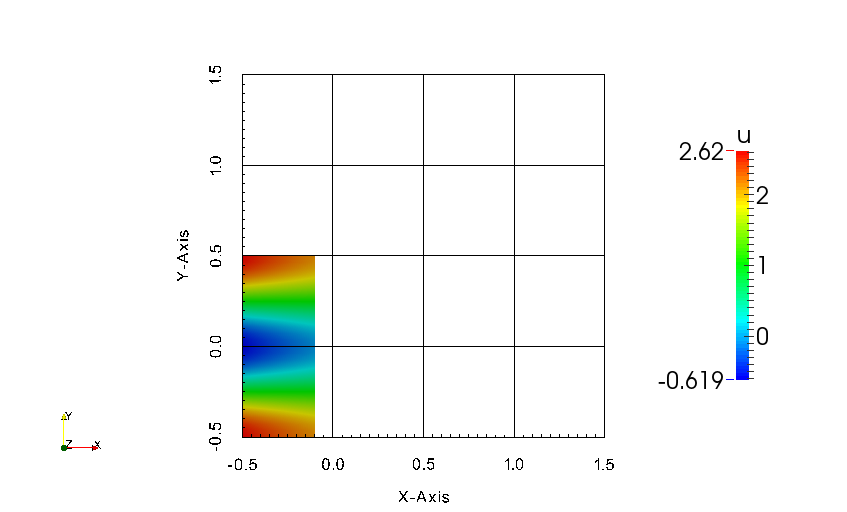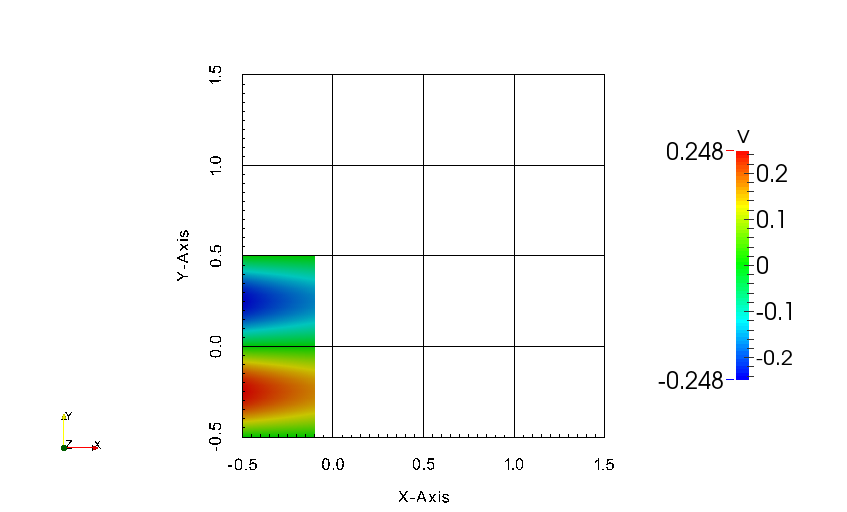Figure 11.5: Velocity profiles for the Kovasznay Flow in truncated domain (2D).

#### 11.10.3 Steady Kovasznay Oseen Flow using the direct solver

In this example, we instead compute the steadhy Kovasznay Oseen flow using the direct solver. In contrast to the velocity correction scheme in which we time-step the solution to the final time, the direct solver computes the solution with a single solve.

##### 11.10.3.1 Input file

We can begin with the same input file as for the previous example, but with the following modifications. For reference, the modified version is provided in the example Oseen_m8.xml.

In the solver information, we must instead select the Steady-Oseen equation type and choose to use the coupled linearised Navier-Stokes

2<I PROPERTY="SolverType"  VALUE="CoupledLinearisedNS" />

Note: Since we are using a coupled system, we are not solving for the pressure. We should therefore remove all references to the variable p in the session. In particular, it should be removed from the EXPANSIONS, VARIABLES, BOUNDARYCONDITIONS and FUNCTIONS sections of the file.

1<FUNCTION NAME="InitialConditions">
2    <E VAR="u" VALUE="0" />
3    <E VAR="v" VALUE="0" />
4</FUNCTION>

We must also provide an advection velocity.

2    <E VAR="u" VALUE="(1-exp(-LAMBDA*x)*cos(2*PI*y))" />
3    <E VAR="v" VALUE="(-LAMBDA/(2*PI))*exp(-LAMBDA*x)*sin(2*PI*y)" />
4</FUNCTION>

##### 11.10.3.2 Running the simulation

Run the simulation using

IncNavierStokesSolver Oseen_m8.xml

The resulting flow field should match the solution from the previous example.

#### 11.10.4 Laminar Channel Flow 2D

In this example, we will simulate the flow through a channel at Reynolds number 1 with fixed boundary conditions.

##### 11.10.4.1 Input file

The input file for this example is given in ChanFlow_m3_SKS.xml. The geometry is a square channel with height and length D = 1, discretised using four quadrilateral elements. We use a quadratic expansion order, which is sufficient to capture the quadratic flow profile. In this example, we choose to use the skew-symmetric form of the advection term. This is chosen in the solver information section:

1<I PROPERTY="EvolutionOperator" VALUE="SkewSymmetric" />

A first-order time integration scheme is used and we set the time-step and number of time integration steps in the parameters section. We also prescribe the kinematic viscosity ν = 1∕Re = 1.

Boundary conditions are defined on the walls (region 0) and at the inflow (regions 1) as Dirichlet for the velocity field and as high-order for the pressure. At the outflow the velocity is left free using Neumann boundary conditions and the pressure is pinned to zero.

1<BOUNDARYCONDITIONS>
2    <REGION REF="0">
3        <D VAR="u" VALUE="0" />
4        <D VAR="v" VALUE="0" />
5        <N VAR="p" USERDEFINEDTYPE="H" VALUE="0" />
6    </REGION>
7    <REGION REF="1">
8        <D VAR="u" VALUE="y*(1-y)" />
9        <D VAR="v" VALUE="0" />
10        <N VAR="p" USERDEFINEDTYPE="H" VALUE="0" />
11    </REGION>
12    <REGION REF="2">
13        <N VAR="u" VALUE="0" />
14        <N VAR="v" VALUE="0" />
15        <D VAR="p" VALUE="0" />
16    </REGION>
17</BOUNDARYCONDITIONS>

Initial conditions are set to zero. The exact solution is a parabolic profile with a pressure gradient dependent on the Reynolds number. This is defined to allow verification of the calculation.

1<FUNCTION NAME="ExactSolution">
2    <E VAR="u" VALUE="y*(1-y)" />
3    <E VAR="v" VALUE="0" />
4    <E VAR="p" VALUE="-2*Kinvis*(x-1)" />
5</FUNCTION>

##### 11.10.4.2 Running the solver
IncNaverStokesSolver ChanFlow_m3_SKS.xml

The error in the solution should be displayed and be close to machine precision

L 2 error (variable u) : 4.75179e-16
L inf error (variable u) : 3.30291e-15
L 2 error (variable v) : 1.12523e-16
L inf error (variable v) : 3.32197e-16
L 2 error (variable p) : 1.12766e-14
L inf error (variable p) : 7.77156e-14

The solution should look similar to that shown in Figure 11.6.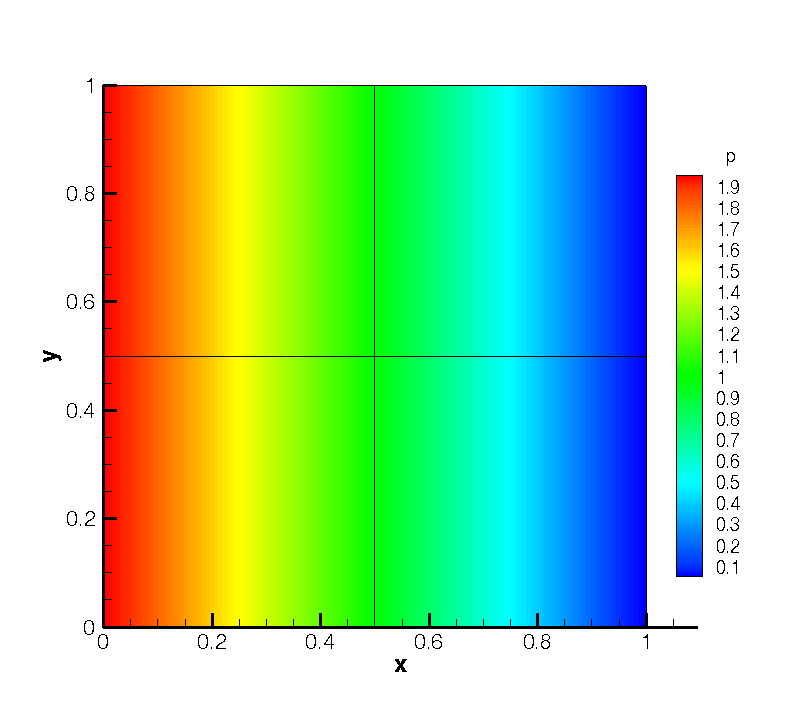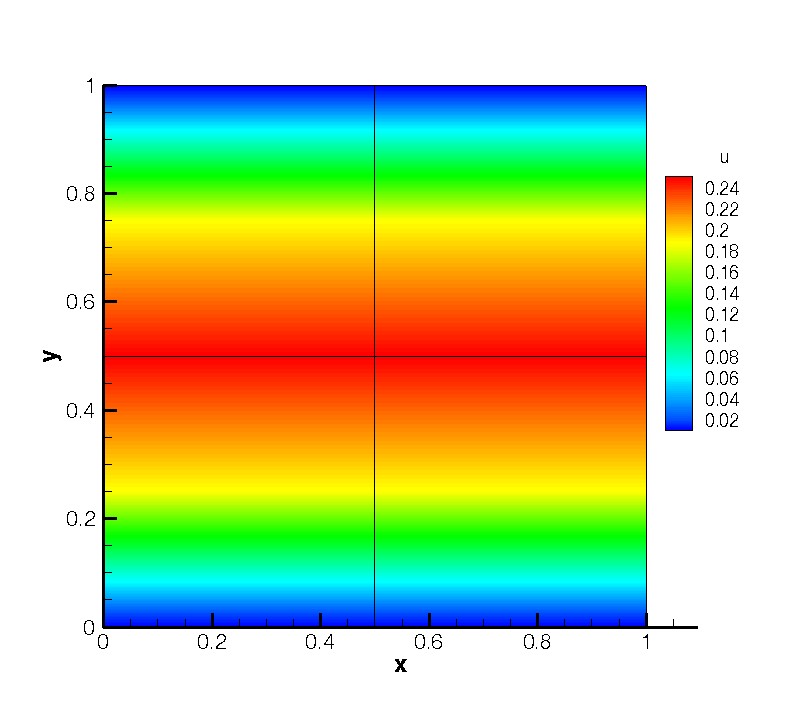Figure 11.6: Pressure and velocity profiles for the laminar channel flow (2D).

#### 11.10.5 Laminar Channel Flow 3D

We now solve the incompressible Navier-Stokes equations on a three-dimensional domain. In particular, we solver the three-dimensional equivalent of the previous example. We will also solve the problem in parallel.

Note: In order to run the example, you must have a version of Nektar++ compiled with MPI. This is the case for the packaged binary distributions.

##### 11.10.5.1 Input file

The input file for this example is given in Tet_channel_m8_par.xml. In this example we use tetrahedral elements, indicated by the A element tags in the geometry section. All dimensions have length D = 1. We will use a 7th-order polynomial expansion. Since we now have three dimensions, and therefore three velocity components, the expansions section is now

1<EXPANSIONS>
2  <E COMPOSITE="C" NUMMODES="8" FIELDS="u,v,w,p" TYPE="MODIFIED" />
3</EXPANSIONS>

The solver information and parameters are similar to the previous example. Boundary conditions must now be defined on the six faces of the domain. Flow is prescribed in the z-direction through imposing a Poiseulle profile on the inlet and side walls. The outlet is zero-Neumann and top and bottom faces impose zero-Dirichlet conditions.

1<BOUNDARYREGIONS>
2    <B ID="0"> C </B>    <!-- Inlet -->
3    <B ID="1"> C </B>    <!-- Outlet -->
4    <B ID="2"> C </B>    <!-- Wall -->
5    <B ID="3"> C </B>    <!-- Wall left -->
6    <B ID="4"> C </B>    <!-- Wall -->
7    <B ID="5"> C </B>    <!-- Wall right -->
8</BOUNDARYREGIONS>
9
10<BOUNDARYCONDITIONS>
11    <REGION REF="0">
12        <D VAR="u" VALUE="0" />
13        <D VAR="v" VALUE="0" />
14        <D VAR="w" VALUE="y*(1-y)" />
15        <N VAR="p" USERDEFINEDTYPE="H" VALUE="0" />
16    </REGION>
17    <REGION REF="1">
18        <N VAR="u" VALUE="0" />
19        <N VAR="v" VALUE="0" />
20        <N VAR="w" VALUE="0" />
21        <D VAR="p" VALUE="0" />
22    </REGION>
23    <REGION REF="2">
24        <D VAR="u" VALUE="0" />
25        <D VAR="v" VALUE="0" />
26        <D VAR="w" VALUE="0" />
27        <N VAR="p" USERDEFINEDTYPE="H" VALUE="0" />
28    </REGION>
29    <REGION REF="3">
30        <D VAR="u" VALUE="0" />
31        <D VAR="v" VALUE="0" />
32        <D VAR="w" VALUE="y*(1-y)" />
33        <N VAR="p" USERDEFINEDTYPE="H" VALUE="0" />
34    </REGION>
35    <REGION REF="4">
36        <D VAR="u" VALUE="0" />
37        <D VAR="v" VALUE="0" />
38        <D VAR="w" VALUE="0" />
39        <N VAR="p" USERDEFINEDTYPE="H" VALUE="0" />
40    </REGION>
41    <REGION REF="5">
42        <D VAR="u" VALUE="0" />
43        <D VAR="v" VALUE="0" />
44        <D VAR="w" VALUE="y*(1-y)" />
45        <N VAR="p" USERDEFINEDTYPE="H" VALUE="0" />
46    </REGION>
47</BOUNDARYCONDITIONS>

Initial conditions and exact solutions are also prescribed.

##### 11.10.5.2 Running the solver

To run the solver in parallel, we use the mpirun command.

mpirun -np 2 IncNaverStokesSolver Tet_channel_m8_par.xml

The expected results are shown in Figure 11.7.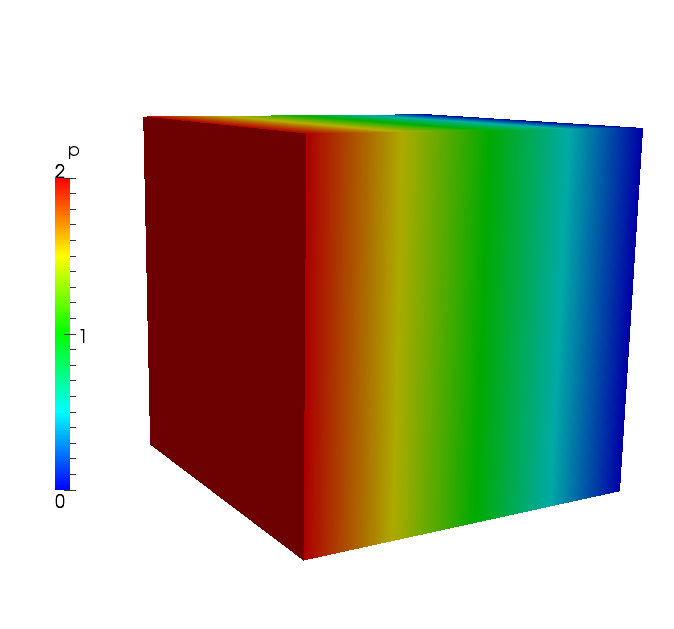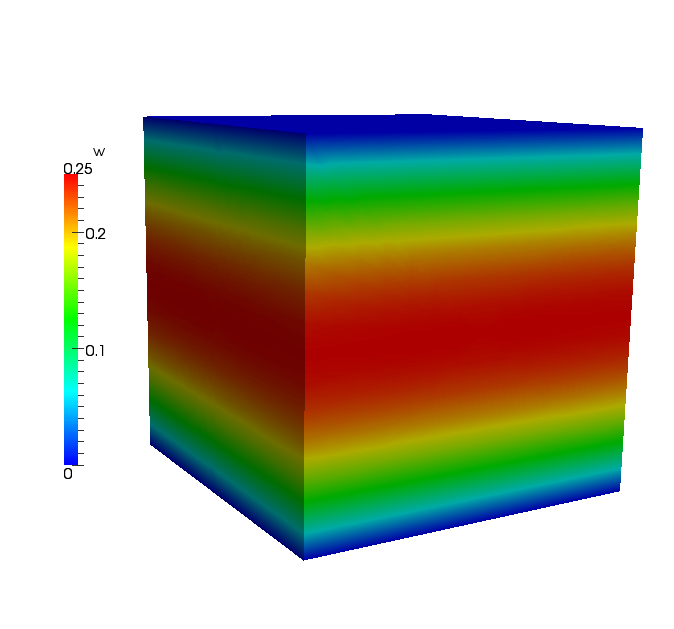Figure 11.7: Pressure and velocity profiles for the laminar channel flow (full 3D).

#### 11.10.6 Laminar Channel Flow Quasi-3D

For domains where at least one direction is geometrically homogeneous, a more efficient discretisation is to use a pure spectral discretisation, such as a Fourier expansion, in these directions. We use this approach to solve the same problem as in the previous example. We reuse the two-dimensional spectral/hp element mesh from the nd couple this with a Fourier expansion in the third component.

##### 11.10.6.1 Input file

The input file for this example is ChanFlow_3DH1D_MVM.xml. We indicate that we are coupling the spectral/hp element domain with a pure spectral expansion using the following solver information

1<I PROPERTY="HOMOGENEOUS" VALUE="1D"/>

We must also specify parameters to describe the particular spectral expansion

1<P> HomModesZ     = 20       </P>
2<P> LZ            = 1.0      </P>

The parameter HomModesZ specifies the number of Fourier modes to use in the homogeneous direction. The LZ parameter specifies the physical length of the domain in that direction.

Note: This example uses an in-built Fourier transform routine. Alternatively, one can use the FFTW library to perform Fourier transforms which typically offers improved performance. This is enabled using the following solver information

1<I PROPERTY="USEFFT" VALUE="FFTW"/>

As with the the spectral/hp element mesh consists of four quadrilateral elements with a second-order polynomial expansion. Since our domain is three-dimensional we have to now include the third velocity component

1<E COMPOSITE="C" NUMMODES="3" FIELDS="u,v,w,p" TYPE="MODIFIED" />

The remaining parameters and solver information is similar to previous examples.

Boundary conditions are specified as for the two-dimensional case (except with the addition of the third velocity component) since the side walls are now implicitly periodic. The initial conditions and exact solution are prescribed as for the fully three-dimensional case.

##### 11.10.6.2 Running the solver
IncNaverStokesSolver ChanFlow_3DH1D_MVM.xml

The results can be post-processed and should match those of the fully three-dimensional case as shown in Figure 11.7.

#### 11.10.7 Turbulent Channel Flow

In this example we model turbulence in a three-dimensional square channel at a Reynolds number of 2000.

Note: This example requires the FFTW Fast-Fourier transform library to be selected when compiling Nektar++.

##### 11.10.7.1 Input file

The input file for this example is TurbChFl_3DH1D.xml. The geometry makes used of the homogeneous extension discussed in the previous example. The channel has height D = 2 and length L = 4π and is discretised using quadratic quadrilateral elements in the spectral/hp element plane and a Fourier basis in the third coordinate direction. The elements are non-uniformly distributed so as to best capture the flow features with fewest degrees of freedom and is shown in Figure 11.8.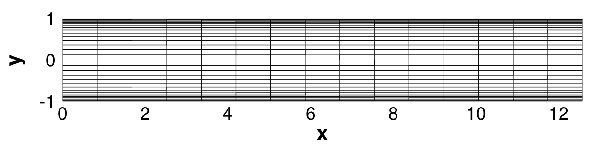Figure 11.8: Mesh used for the turbulent channel flow.

The spanwise length of the channel is set using the LZ parameter and discretised with 32 Fourier modes by setting the value of HomModesZ.

1<P> HomModesZ     = 32          </P>
2<P> LZ            = 4*PI/3     </P>

A second-order IMEX scheme is used for time-integration scheme is used with a time-step of 0.0001. The length of the simulation is 1 time-unit (10,000 steps).

Periodicity is naturally enforced in the spanwise direction, so boundary conditions need only be provided for the upper and lower walls, inlet and outlet as denoted by the following BOUNDARYREGIONS.

1<BOUNDARYREGIONS>
2  <B ID="0"> C </B> //walls
3  <B ID="1"> C </B> //inflow
4  <B ID="2"> C </B> //outflow
5</BOUNDARYREGIONS>

In this example, we will use a body force to drive the flow and so, in addition to the spanwise periodicity, enforce periodicity in the streamwise direction of the spectral/hp element mesh. This is achieved by imposing the following boundary conditions

1  <REGION REF="1">
2    <P VAR="u" VALUE="" />
3    <P VAR="v" VALUE="" />
4    <P VAR="w" VALUE="" />
5    <P VAR="p" VALUE="" />
6  </REGION>
7  <REGION REF="2">
8    <P VAR="u" VALUE="" />
9    <P VAR="v" VALUE="" />
10    <P VAR="w" VALUE="" />
11    <P VAR="p" VALUE="" />
12  </REGION>

Here, we use P to denote the boundary type is periodic, and the value in square brackets denotes the boundary region to which the given boundary is periodic with. In this case regions 1 and 2 are denoted periodic with each other.

A streamwise plug-profile initial condition is prescribed such that u = 1 everywhere, except the wall boundaries. The body force requires two components in the XML file. The first specifies the type of forcing to apply and appears directly within the NEKTAR tag.

1<FORCING>
2    <FORCE TYPE="Body">
3        <BODYFORCE> BodyForce </BODYFORCE>
4    </FORCE>
5</FORCING>

The second defines the BodyForce function which will be used and is located within the CONDITIONS section,

1<FUNCTION NAME="BodyForce">
2    <E VAR="u" VALUE="2*Kinvis" />
3    <E VAR="v" VALUE="0" />
4    <E VAR="w" VALUE="0" />
5</FUNCTION>

To improve numerical stability, we also enable dealising of the advection term. This uses additional points to perform the quadrature and then truncates the higher-order terms when projecting back onto the polynomial space, thereby removing spurious oscillations. It is enabled by setting the solver information tag

1<I PROPERTY="DEALIASING" VALUE="True" />

This feature is only available when using the FFTW library is used, so we enable this using

1<I PROPERTY="USEFFT" VALUE="FFTW" />
##### 11.10.7.2 Running the solver

To run the solver, we use the following command

IncNaverStokesSolver TurbChFl_3DH1D.xml

The result after transition has occurred is illustrated in Figure 11.9.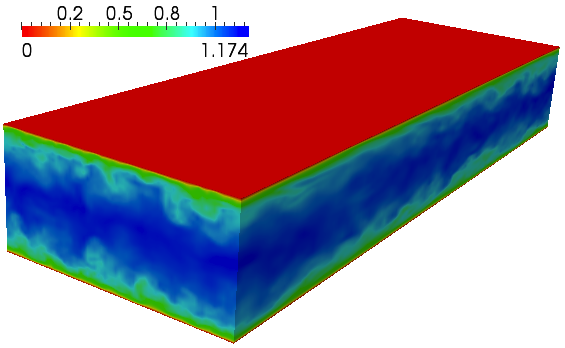Figure 11.9: Velocity profile of the turbulent channel flow (quasi-3D).

#### 11.10.8 Turbulent Pipe Flow

In this example we simulate flow in a pipe at Reynolds number 3000 using a mixed spectral/hp element and Fourier discretisation. The Fourier expansion is used in the streamwise direction in this case and the spectral/hp elements are used to capture the circular cross-section.

##### 11.10.8.1 Input File

The circular pipe has diameter D = 1, the 2D mesh is composed of 64 quadrilateral elements and the streamwise direction is discretised with 128 Fourier modes. An illustrative diagram of the discretisation is given in Figure 11.10.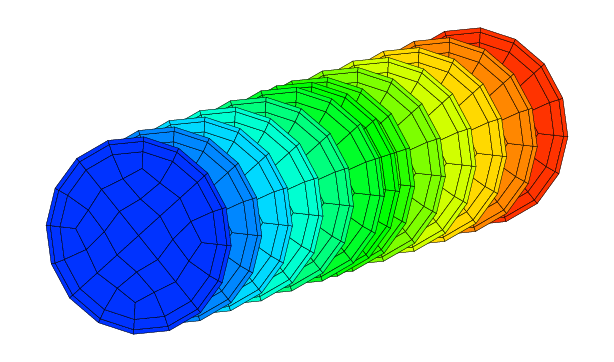Figure 11.10: Domain for the turbulent pipe flow problem.

The input file for this example is Pipe_turb.xml. We use 7th-order lagrange polynomials through the Gauss-Lobatto-Legendre points for the quadrilateral expansions.

1<E COMPOSITE="C" NUMMODES="8" FIELDS="u,v,w,p" TYPE="GLL_LAGRANGE_SEM" />

We set the Fourier options, as in the previous example, except using 128 modes and a length of 5 non-dimensional units. A small amplitude noise is also added to the initial condition, which is a plug profile, to help stimulate transition. Since the streamwise direction is the Fourier direction, we must necessarily use a body force to drive the flow.

##### 11.10.8.2 Running the solver

In this example we will run the solver in parallel. Due to the large number of Fourier modes and relatively few elements, it is more efficient to parallelise in the streamwise direction. We can specify this by providing an additional flag to the solver, –npz. This indicates the number of partitions in the z-coordinate. In this example, we will only run two processes. We therefore would specify –npz 2 to ensure parallelisation only occurs in the Fourier direction.

To improve the efficiency of the solver further, we would prefer to solve the Helmholtz problems within the spectral/hp element planes using a direct solver (since no parallelisation is necessary). The default when running in parallel is to use an iterative solver, so we explicitly specify the type of algorithm to use in the session file solver information:

1<I PROPERTY="GlobalSysSoln" VALUE="DirectStaticCond" />

The solver can now be run as follows

mpirun -np 2 IncNavierStokesSolver --npz 2 Pipe_turb.xml

When the pipe transitions, the result should look similar to Figure 11.11.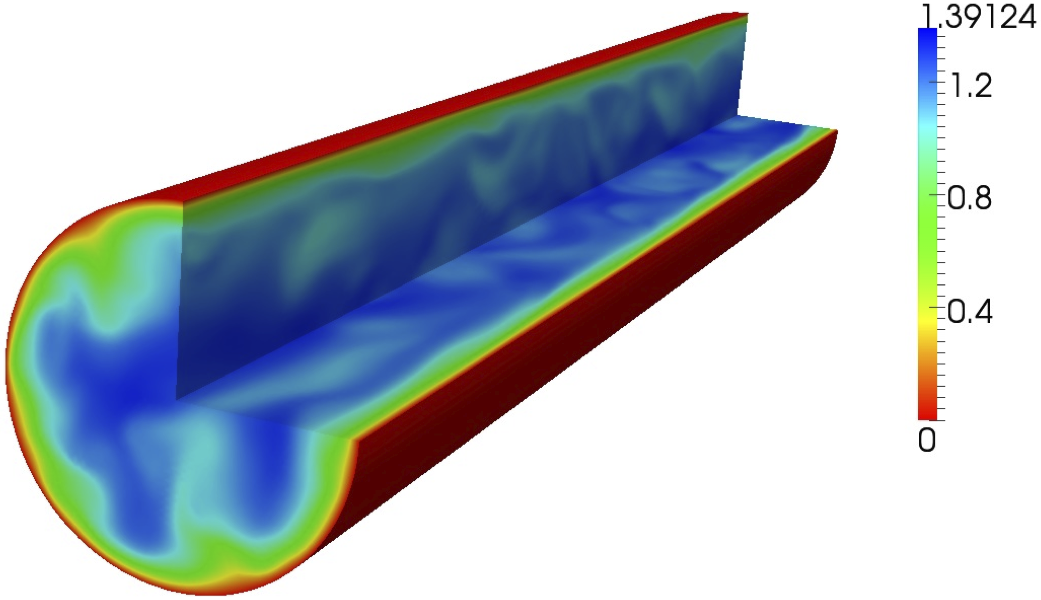Figure 11.11: Velocity profile of the turbulent pipe flow (quasi-3D).

#### 11.10.9 Aortic Blood Flow

The following example demonstrates the application of the incompressible Navier-Stokes solver using the Velocity Correction Scheme algorithm for modelling viscid Newtonian blood flow in a region of a rabbit descending thoracic aorta with intercostal branch pairs. Such studies are necessary to understand the effect local blood flow changes have on cardiovascular diseases such as atherosclerosis.

In the following we will numerically solve for the three dimensional velocity and pressure field for steady boundary conditions. The Reynolds number under consideration is 300, which is physiologically relevant.

Geometry

The geometry under consideration is a segment of a rabbit descending aorta with two pairs of intercostal arteries branching off. The inlet has a diameter D = 3.32mm.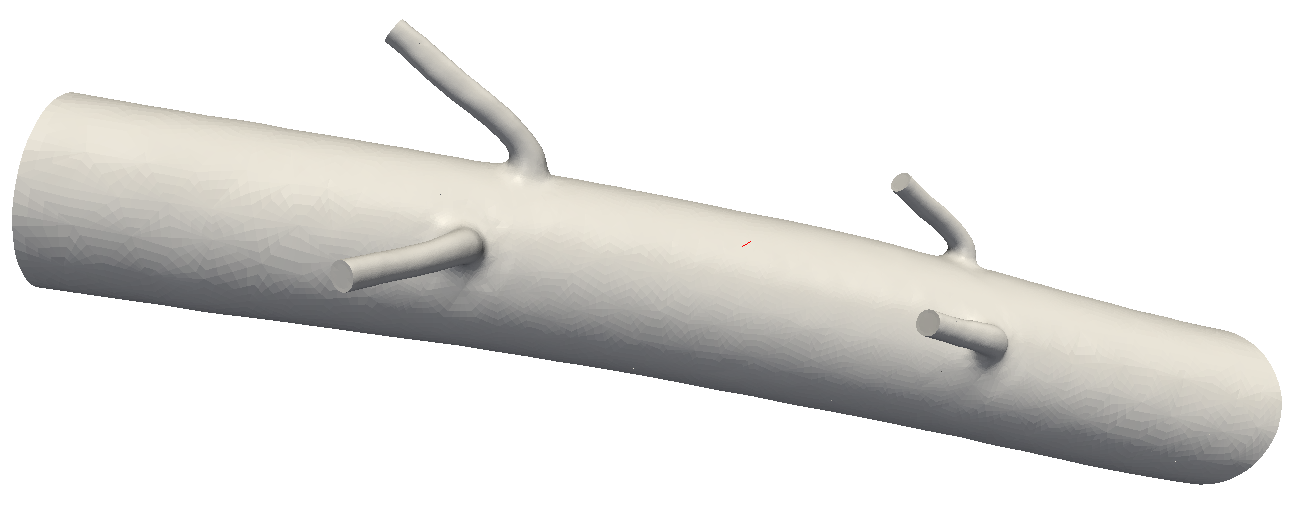Figure 11.12: Reduced region of rabbit descending thoracic aorta.

In order to capture the physics of the flow in the boundary layer, a thin layer consisting of prismatic elements is created adjacent to the surface, and curved using spherigons. The interior consist of tetrahedral elements.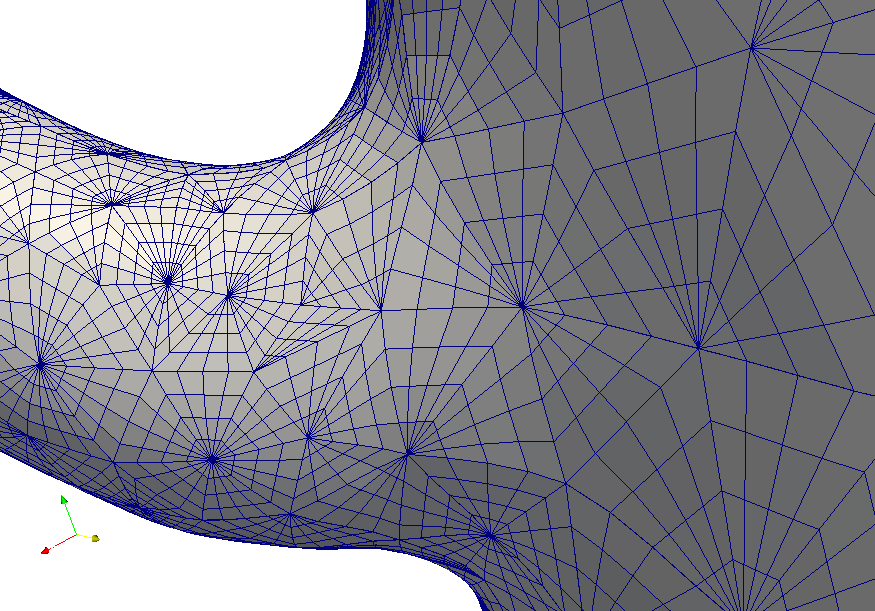Figure 11.13: Surface mesh indicating curved surface elements at a branch location.

Input parameters

##### 11.10.9.1 Expansion:

In this example we will use a fourth order polynomial expansion. There are two composites defined here since we have both prismatic and tetrahedral elements.

1<EXPANSIONS>
2    <E COMPOSITE="C" NUMMODES="5" TYPE="MODIFIED" FIELDS="u,v,w,p" />
3    <E COMPOSITE="C" NUMMODES="5" TYPE="MODIFIED" FIELDS="u,v,w,p" />
4</EXPANSIONS>

##### 11.10.9.2 Time Integration Scheme information:
1<TIMEINTEGRATIONSCHEME>
2    <METHOD> IMEX </METHOD>
3    <ORDER> 1 </ORDER>
4</TIMEINTEGRATIONSCHEME>

##### 11.10.9.3 Solver information:
1<SOLVERINFO>
2    <I PROPERTY="SolverType"            VALUE="VelocityCorrectionScheme" />
5    <I PROPERTY="Projection"            VALUE="Galerkin"                 />
6</SOLVERINFO>

##### 11.10.9.4 Parameters:

Since we are prescribing a Reynolds number of 300, and to simplify the problem definition, we set the mean inlet velocity to 1, this allows us to define the kinematic viscosity as ν =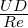=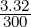= 1∕90.36.

1<PARAMETERS>
2    <P> TimeStep       = 0.0005     </P>
3    <P> NumSteps       = 1600       </P>
4    <P> IO_CheckSteps  = 200        </P>
5    <P> IO_InfoSteps   = 50         </P>
6    <P> Kinvis         = 1.0/90.36  </P>
7</PARAMETERS>

##### 11.10.9.5 Boundary conditions:

For the purpose of this example a blunted inlet velocity profile has been prescribed. Ideally to obtain more significant results, the velocity profile at the inlet would be obtained from previous simulations on the complete rabbit aorta (including aortic root, aortic arch, and descending aorta with all 5 pairs of intercostal arteries), where a blunted profile at the aortic root is a better representation of reality.

Dirichlet boundary conditions are imposed for the velocity at the inlet, as well as on the wall to account for the no-slip condition. Neumann boundary conditions are imposed for the velocity field at the outlets where fully developed flow is imposed.

1<BOUNDARYREGIONS>
2    <B ID="0"> C </B>          <!-- Inlet -->
3    <B ID="1"> C[3,4,5,6] </B>    <!-- intercostal outlets -->
4    <B ID="2"> C </B>          <!-- outlet -->
5    <B ID="3"> C </B>          <!-- wall -->
6</BOUNDARYREGIONS>
7
8<BOUNDARYCONDITIONS>
9    <REGION REF="0">
10        <D VAR="u" VALUE="0.024" />
11        <D VAR="v" VALUE="-0.064" />
12        <D VAR="w" VALUE="-0.998" />
13        <N VAR="p" USERDEFINEDTYPE="H" VALUE="0" />
14    </REGION>
15    <REGION REF="1">
16        <N VAR="u" VALUE="0" />
17        <N VAR="v" VALUE="0" />
18        <N VAR="w" VALUE="0" />
19        <D VAR="p" VALUE="0" />
20    </REGION>
21    <REGION REF="2">
22        <N VAR="u" VALUE="0" />
23        <N VAR="v" VALUE="0" />
24        <N VAR="w" VALUE="0" />
25        <D VAR="p" VALUE="0" />
26    </REGION>
27    <REGION REF="3">
28        <D VAR="u" VALUE="0" />
29        <D VAR="v" VALUE="0" />
30        <D VAR="w" VALUE="0" />
31        <N VAR="p" USERDEFINEDTYPE="H" VALUE="0" />
32    </REGION>
33</BOUNDARYCONDITIONS>

##### 11.10.9.6 Functions:
1<FUNCTION NAME="InitialConditions">
2    <E VAR="u" VALUE="0" />
3    <E VAR="v" VALUE="0" />
4    <E VAR="w" VALUE="0" />
5    <E VAR="p" VALUE="0" />
6</FUNCTION>

##### 11.10.9.7 Results

We can visualise the internal velocity field by applying a volume render filter in ParaView.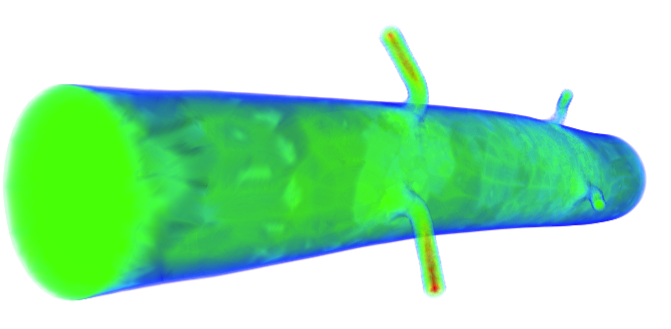Figure 11.14: The solved-for velocity field.

It is possible to visualise the wall shear stress distribution by running the FldAddWSS utility.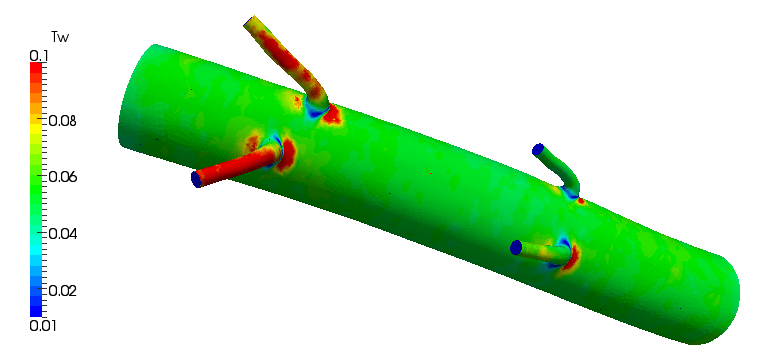Figure 11.15: Non-dimensional wall shear stress distribution.

#### 11.10.10 finite-strip modeling of flow past flexible cables

As a computationally efficient model, strip theory-based modeling technique has been proposed previously to predict vortex-induced vibration (VIV) for higher Reynolds number flows. In the strip theory-based model, the fluid flow solution is obtained on a series of 2D computational planes (also called as “strips”) along the riser’s axis direction. These strips then are coupled with each other through structural dynamic model of the riser, and then VIV response prediction is achieved by the strip-structure interactions.In the 2D strip theory, it is assumed that the flow is purely two-dimensional without spanwise correlation, which allows the problems to be split into various 2D planes. A consequence of 2D strip solution under this assumption is that it is unable to reflect the influence of spanwise wake turbulence on the structural dynamics. In order to overcome this shortcoming, we proposed a new module in the framework of Nektar++, in which a spanwise scale is locally allocated to each one of the strips, so that the spanwise velocity correlation is reconstructed in the flow field within each strips. In particular, this model lets the fluid domain to be divided in N strips with thickness ratio of Lz∕D and evenly distributed along the spanwise (z) direction. The gap between the neighboring strips, represented by Lg, satisfies relation Lc = N(Lz + Lg). Since the strip in this model has finite scale in the z-direction, we named it as finite strip to distinguish from traditional 2D strip plane. Next, the flow dynamics within each individual strips are modeled by viscous incompressible Navier-Stokes equations, while a tensioned beam model is employed to govern the dynamics of the flexible structures. In this example, we will show how to perform a finite-strip model to predict the vortex-induced vibration responses of flexible cables. Let us consider a vortex-induced vibration of a slender cable with an aspect ratio of Lz∕D=4π, which is immersed in uniform flows at Re=100.

##### 11.10.10.1 Input File

The cable with a mass ratio (defined as the ratio of the total oscillating mass to the mass of displaced fluid) of 1 has diameter D = 1, the 2D mesh is composed of 284 quadrilateral elements. The spanwise direction is split in 16 strips with thickness ratio of Lc∕D=π/8 and one pair of complex Fourier modes for each one of the strips. We will use a sixth order polynomial expansion for the spectral element and the input file for this example is CylFlow_HomoStrip.xml.

1<E COMPOSITE="C" NUMMODES="6" TYPE="MODIFIED" FIELDS="u,v,w,p" />

To use the finite strip routines we need just to insert a flag of "HomoStrip" in the solver information as below, in addition, we need to specify the types of vibration and support ends for the cables. In this case, the vibration type is specified as VALUE="CONSTRAINED", which means that the cable’s vibration is constrained only in the crossflow direction. Other options include VALUE="FREE" and "FORCED", respectively corresponding to the free vibrations in both streamwise and crossflow directions and forced vibration by specified functions given in input file. For the support ends of the cable, another option of VALUE="PINNED-PINNED" is available for the simulations, which satisfies the condition of zero values of displacements on the support ends.

##### 11.10.10.2 Solver information:
1  <SOLVERINFO>
2      <I PROPERTY="HomoStrip"     VALUE="True"        />
3      <I PROPERTY="VibrationType" VALUE="CONSTRAINED" />
4      <I PROPERTY="SupportType"   VALUE="FREE-FREE"   />
5  </SOLVERINFO>

##### 11.10.10.3 Parameters

All the simulation parameters are specified in the section as follows.

1  <PARAMETERS>
2      <P> LZ            = PI/8             </P> <!--thickness ratio-->
3      <P> LC            = 4*PI             </P> <!--aspect ratio-->
4      <P> A             = 0.025            </P>
5      <P> omega         = 1.0              </P>
6      <P> PROC_Z        = 16               </P>
7      <P> Strip_Z       = 16               </P> <!--number of the strips-->
8      <P> DistStrip     = PI/4             </P> <!--distance of the strips-->
9      <P> StructStiff   = 0.02             </P>
10      <P> StructRho     = 2.0              </P>
11      <P> CableTension  = 8.82             </P>
12      <P> BendingStiff  = 0.0              </P>
13      <P> FictDamp      = 0.0              </P>
14      <P> FictMass      = 3.0              </P>
15  </PARAMETERS>
16

##### 11.10.10.4 Running the solver

In this example we will run the solver in parallel. We can specify the number of the strips by providing an additional flag to the solver, –nsz. In this example, we will run 16 strips, therefore it would be specified as –nsz 16. The solver can now be run as follows

mpirun -np 16 IncNavierStokesSolver  CylFlow_HomoStrip.xml --npz 16 --nsz 16

The simulation results are illustrated in spanwise vorticity contours in Figure 11.16. The wake response of the cable appears as standing wave pattern in the earlier stage and then it transitions into travelling wave response, as shown in this figure.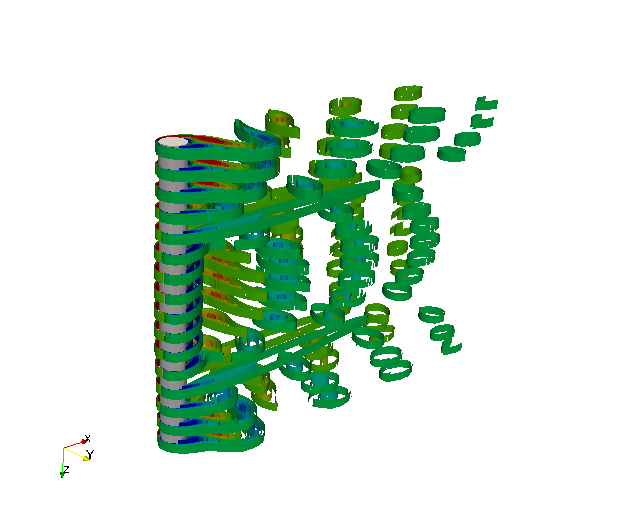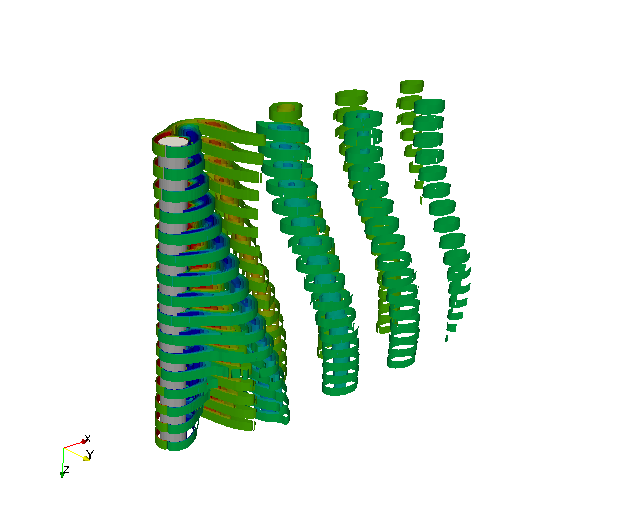Figure 11.16: Spanwise vorticity contours in standing wave and travelling wave patterns predicted in finite strip modeling.

#### 11.10.11 2D direct stability analysis of the channel flow

In this example, it will be illustrated how to perform a direct stability analysis using Nektar++. Let us consider a canonical stability problem, the flow in a channel which is confined in the wall-normal direction between two infinite plates (Poiseuille flow) at Reynolds number 7500. This problem is a particular case of the stability solver for the IncNavierStokesSolver.

##### 11.10.11.1 Background

We consider the linearised Incompressible Navier-Stokes equations: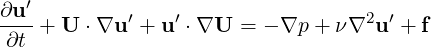(11.39a)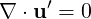(11.39b)

We are interested to compute the leading eigenvalue of the system using the Arnoldi method.

##### 11.10.11.2 Geometry

The geometry under consideration is a 2D channel.

##### 11.10.11.3 Mesh Definition

In the GEOMETRY section, the dimensions of the problem are defined. Then, the coordinates (XSCALE, YSCALE, ZSCALE) of each vertices of each element are specified. As this input file defines a two-dimensional problem: ZSCALE = 0.

1<GEOMETRY DIM="2" SPACE="2">
2        <VERTEX>
3            <V ID="0">3.142e+00 1.000e+00 0.000e+00</V>
4            ...
5            <V ID="62">-3.142e+00 -1.000e+00 0.000e+00</V>
6        </VERTEX>

Edges can now be defined by two vertices.

1<EDGE>
2            <E ID="0">    0  1   </E>
3            ...
4            <E ID="109">   62  55   </E>
5        </EDGE>

In the ELEMENT section, the tag T and Q define respectively triangular and quadrilateral element. Triangular elements are defined by a sequence of three edges and quadrilateral elements by a sequence of four edges.

1        <ELEMENT>
2            <Q ID="0">    0     1     2     3 </Q>
3            ...
4            <Q ID="47">  107   108   109    95 </Q>
5        </ELEMENT>
6

Finally, collections of elements are listed in the COMPOSITE section and the DOMAIN section specifies that the mesh is composed by all the triangular and quadrilateral elements. The other composites will be used to enforce boundary conditions.

1              <COMPOSITE>
2            <C ID="0"> Q[0-47] </C>
3            <C ID="1"> E[17,31,44,57,70,83,96,109,0,19,32,45,58,71,84,97] </C> //wall
4            <C ID="2"> E[3,6,9,12,15,18] </C>//inflow
5            <C ID="3"> E[98,100,102,104,106,108] </C> //outflow
6        </COMPOSITE>
7        <DOMAIN> C </DOMAIN>
8</GEOMETRY>
9

##### 11.10.11.4 Expansion

This section defines the polynomial expansions used on each composites. For this example we will use a 10th order polynomial, i.e. P = 11.

1<EXPANSIONS>
2     <E COMPOSITE="C" NUMMODES="11" FIELDS="u,v,p" TYPE="MODIFIED" />
3</EXPANSIONS>
4

##### 11.10.11.5 Time Integration Scheme

For this scheme, an implicit-explicit time-integration scheme is used and we choose one of first order.

1  <TIMEINTEGRATIONSCHEME>
2    <METHOD> IMEX </METHOD>
3    <ORDER> 1 </ORDER>
4  </TIMEINTEGRATIONSCHEME>
5

##### 11.10.11.6 Solver Info

In this example the EvolutionOperator must be Direct to consider the linearised Navier-Stokes equations and the Driver was set up to ModifiedArnoldi for the solution of the eigenproblem.

1  <SOLVERINFO>
2    <I PROPERTY="SolverType"            VALUE="VelocityCorrectionScheme" />
4    <I PROPERTY="EvolutionOperator"     VALUE="Direct"                   />
5    <I PROPERTY="Projection"            VALUE="Galerkin"                 />
6    <I PROPERTY="Driver"                VALUE="ModifiedArnoldi"         />
7  </SOLVERINFO>
8

##### 11.10.11.7 Parameters

All the stability parameters are specified in this section.

1<PARAMETERS>
2      <P> TimeStep      = 0.002 </P>
3      <P> NumSteps      = 500 </P>
4      <P> IO_CheckSteps = 1000    </P>
5      <P> IO_InfoSteps  = 10    </P>
6      <P> Re            = 7500     </P>
7      <P> Kinvis         =1./Re   </P>
8      <P> kdim           =16   </P>
9      <P> nvec           =2   </P>
10      <P> evtol          =1e-5</P>
11      <P> nits           =5000 </P>
12   </PARAMETERS>
13

##### 11.10.11.8 Boundary Conditions
1 <BOUNDARYREGIONS>
2            <B ID="0"> C </B>
3            <B ID="1"> C </B>
4            <B ID="2"> C </B>
5        </BOUNDARYREGIONS>
6
7        <BOUNDARYCONDITIONS>
8            <REGION REF="0">
9                <D VAR="u" VALUE="0" />
10                <D VAR="v" VALUE="0" />
11                <N VAR="p" USERDEFINEDTYPE="H" VALUE="0" />
12            </REGION>
13            <REGION REF="1">
14                <P VAR="u" VALUE="" />
15                <P VAR="v" VALUE="" />
16                <P VAR="p" VALUE="" />
17            </REGION>
18            <REGION REF="2">
19                <P VAR="u" VALUE="" />
20                <P VAR="v" VALUE="" />
21                <P VAR="p" VALUE="" />
22            </REGION>
23        </BOUNDARYCONDITIONS>
24

##### 11.10.11.9 Function

We need to set up the base flow that can be specified as a function BaseFlow. In case the base flow is not analytical, it can be generated by means of the Nonlinear evolution operator using the same mesh and polynomial expansion. The initial guess is specified in the InitialConditions functions and can be both analytical or a file. In this example it is read from a file.

1<FUNCTION NAME="BaseFlow">
2            <F VAR="u,v,p" FILE="ChanStability.bse" />
3        </FUNCTION>
4
5        <FUNCTION NAME="InitialConditions">
6            <F VAR="u,v,p" FILE="ChanStability.rst" />
7        </FUNCTION>
8

If the ModifiedArnoldi driver is used, a subregion of the computational domain can be used to calculate the eigenvalues and eigenvectors by defining an unmask function. This can be used to improve the accuracy and to accelerate convergence if the computational domain is large and contains very coarse elements.

2    <E VAR="C0" VALUE=" x" />
3    <E VAR="C1" VALUE="-x+1." />
4    <E VAR="C2" VALUE=" y+1.5" />
5    <E VAR="C3" VALUE="-y+1.5" />
6</FUNCTION>
7
9    <E VAR="C0" VALUE=" x" />
10    <E VAR="C1" VALUE="-x+1." />
11    <E VAR="C2" VALUE=" y+1.5" />
12    <E VAR="C3" VALUE="-y+1.5" />
13</FUNCTION>
14
15...

Each function Unmask0 selects elements whose center satisfies C0>0 and C1>0 and C2>0, and so on. More than one unmasked function can be defined, and the finally selected region is the union of all unmasked regions.

##### 11.10.11.11 Usage

IncNavierStokesSolver ChanStability.xml

##### 11.10.11.12 Results

The stability simulation takes about 250 iterations to converge and the dominant eigenvalues (together with the respective eigenvectors) will be printed. In this case it was found λ1,2 = 1.000224 ×e±0.24984i. Therefore, since the magnitude of the eigenvalue is larger than 1, the flow is absolutely unstable. It is possible to visualise the eigenvectors using the post-processing utilities. The figure shows the profile of the two eigenmode component, which shows the typical Tollmien - Schlichting waves that arise in viscous boundary layers.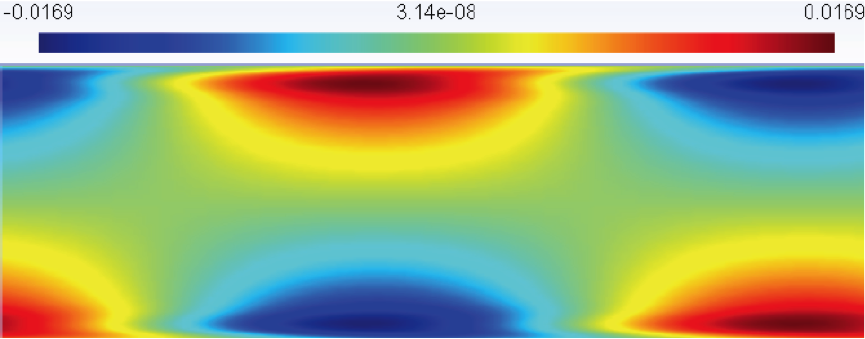Figure 11.17: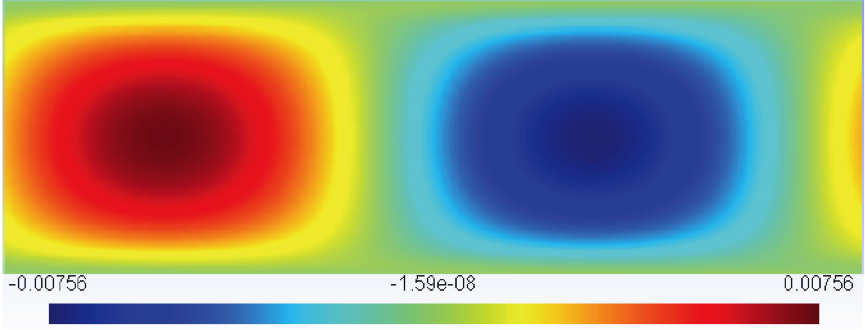Figure 11.18:

#### 11.10.12 2D adjoint stability analysis of the channel flow

In this example, it will be illustrated how to perform an adjoint stability analysis using Nektar++. Let us consider a canonical stability problem, the flow in a channel which is confined in the wall-normal direction between two infinite plates (Poiseuille flow) at Reynolds number 7500

##### 11.10.12.1 Background

We consider the equations: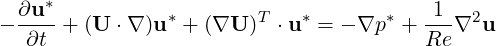(11.40a)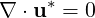(11.40b)

We are interested in computing the leading eigenvalue of the system using the Arnoldi method.

##### 11.10.12.2 Geometry & Mesh

The geometry and mesh are the same ones used for the direct stability analysis in the previous example.

##### 11.10.12.3 Time Integration Scheme

For this scheme, an implicit-explicit time-integration scheme is used and we choose one of first order.

1  <TIMEINTEGRATIONSCHEME>
2    <METHOD> IMEX </METHOD>
3    <ORDER> 1 </ORDER>
4  </TIMEINTEGRATIONSCHEME>
5

##### 11.10.12.4 Solver Info

This sections defines the problem solved. In this example the EvolutionOperator must be Adjoint to consider the adjoint Navier-Stokes equations and the Driver was set up to ModifiedArnoldi for the solution of the eigenproblem.

1
2<SOLVERINFO>
3      <I PROPERTY="SolverType"            VALUE="VelocityCorrectionScheme" />
6      <I PROPERTY="Projection"            VALUE="Galerkin"                 />
7      <I PROPERTY="Driver"                VALUE= "ModifiedArnoldi"         />
8</SOLVERINFO>
9  \end{subequations}
10
11\textbf{Parameters}
12
13    \begin{lstlisting}[style=XMLStyle]
14<PARAMETERS>
15      <P> TimeStep      = 0.002 </P>
16      <P> NumSteps      = 500 </P>
17      <P> IO_CheckSteps = 1000    </P>
18      <P> IO_InfoSteps  = 10    </P>
19      <P> Re            = 7500     </P>
20      <P> Kinvis         =1./Re   </P>
21      <P> kdim           =16   </P>
22      <P> nvec           =2   </P>
23      <P> evtol          =1e-5</P>
24      <P> nits           =5000 </P>
25</PARAMETERS>
26

##### 11.10.12.5 Boundary Conditions
1 <BOUNDARYREGIONS>
2            <B ID="0"> C </B>
3            <B ID="1"> C </B>
4            <B ID="2"> C </B>
5        </BOUNDARYREGIONS>
6
7        <BOUNDARYCONDITIONS>
8            <REGION REF="0">
9                <D VAR="u" VALUE="0" />
10                <D VAR="v" VALUE="0" />
11                <N VAR="p" USERDEFINEDTYPE="H" VALUE="0" />
12            </REGION>
13            <REGION REF="1">
14                <P VAR="u" VALUE="" />
15                <P VAR="v" VALUE="" />
16                <P VAR="p" VALUE="" />
17            </REGION>
18            <REGION REF="2">
19                <P VAR="u" VALUE="" />
20                <P VAR="v" VALUE="" />
21                <P VAR="p" VALUE="" />
22            </REGION>
23        </BOUNDARYCONDITIONS>
24

##### 11.10.12.6 Functions

We need to set up the base flow that can be specified as a function BaseFlow. In case the base flow is not analytical, it can be generated by means of the Nonlinear evolution operator using the same mesh and polynomial expansion.

1        <FUNCTION NAME="BaseFlow">
2            <F VAR="u,v,p" FILE="ChanStability.bse" />
3        </FUNCTION>
4

The initial guess is specified in the InitialConditions functions and can be both analytical or a file. In this example it is read from a file.

1        <FUNCTION NAME="InitialConditions">
2            <F VAR="u,v,p" FILE="ChanStability.rst" />
3        </FUNCTION>
4

##### 11.10.12.7 Usage

IncNavierStokesSolver ChanStability_adj.xml

##### 11.10.12.8 Results

The equations will then be evolved backwards in time (consistently with the negative sign in front of the time derivative) and the leading eigenvalues will be computed after about 300 iterations. It is interesting to note that their value is the same one computed for the direct problem, but the eigenmodes present a different shape.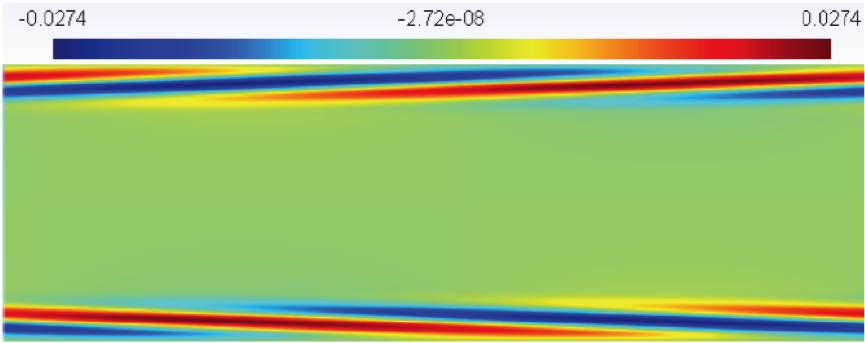Figure 11.19: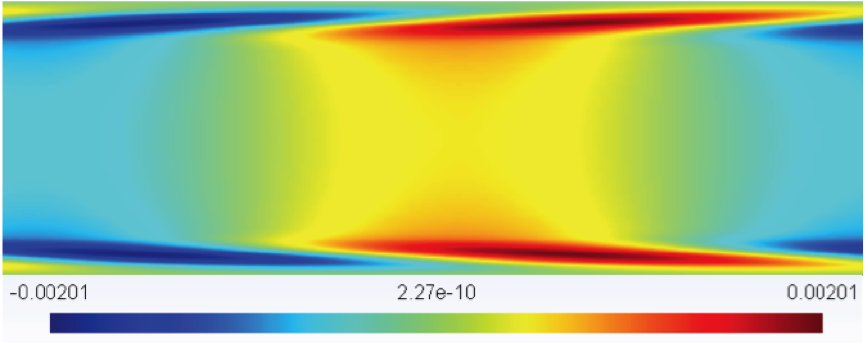Figure 11.20:

#### 11.10.13 2D Transient Growth analysis of a flow past a backward-facing step

In this section it will be described how to perform a transient growth stability analysis using Nektar++. Let us consider a flow past a backward-facing step (figure 11.21). This is an important case because it allows us to understand the effects of separation caused by abrupt changes in the geometry and it is a common geometry in several studies of flow control and turbulence of separated flow.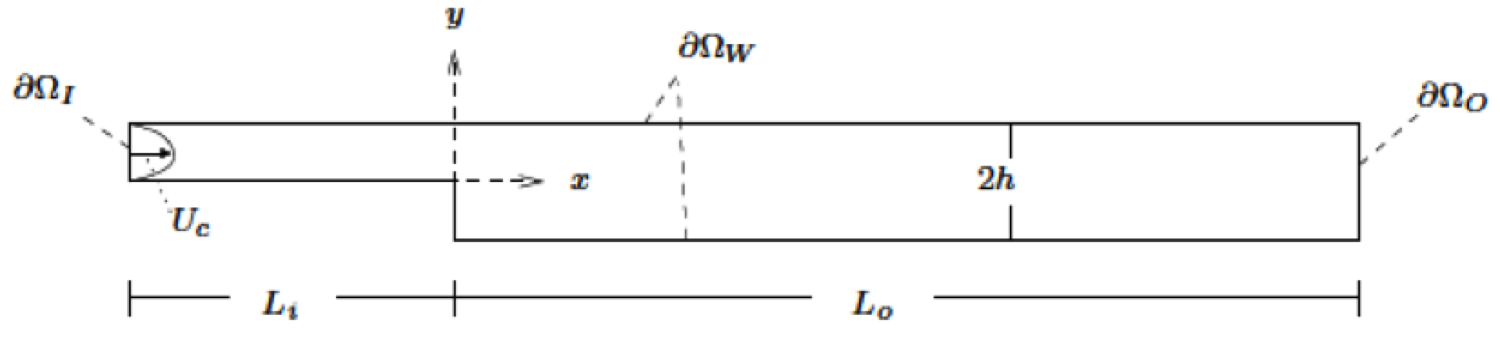Figure 11.21:

##### 11.10.13.1 Background

Transient growth analysis allows us to study the presence of convective instabilities that can arise in stable flows. Despite the fact that these instabilities will decay for a long time (due to the stability of the flow), they can produce significant increases in the energy of perturbations. The phenomenon of transient growth is associated with the non-normality of the linearised Navier-Stokes equations and it consists in computing the perturbation that leads to the highest energy growth for a fixed time horizon.

##### 11.10.13.2 Input Parameters

In the GEOMETRY section, the dimensions of the problem are defined. Then, the coordinates (XSCALE, YSCALE, ZSCALE) of each vertices are specified. As this input file defines a two-dimensional problem: ZSCALE = 0.

1<GEOMETRY DIM="2" SPACE="2">
2   <VERTEX>
3       <V ID="0">3.000e+00 -1.000e+00 0.000e+00</V>
4       ...
5       <V ID="399">-1.000e+01 0.000e+00 0.000e+00</V>
6   </VERTEX>
7

Edges can now be defined by two vertices.

1<EDGE>
2       <E ID="0">    0  1   </E>
3       ...
4       <E ID="828">  399  394   </E>
5   </EDGE>
6

In the ELEMENT section, the tag T and Q define respectively triangular and quadrilateral element. Triangular elements are defined by a sequence of three edges and quadrilateral elements by a sequence of four edges.

1<ELEMENT>
2       <T ID="0">    0     1     2 </T>
3       ...
4       <T ID="209">  333   314   332 </T>
5       <Q ID="210">  334   335   336     0 </Q>
6       ...
7       <Q ID="429">  826   827   828   818 </Q>
8   </ELEMENT>
9

Finally, collections of elements are listed in the COMPOSITE section and the DOMAIN section specifies that the mesh is composed by all the triangular and quadrilateral elements. The other composites will be used to enforce boundary conditions.

1<COMPOSITE>
2       <C ID="0"> T[0-209] </C>
3       <C ID="1"> Q[210-429] </C>
4       <C ID="2"> E[2-3,7,10,16,21,2,...,828] </C>
5       <C ID="3"> E[821,823,825,827] </C>
6       <C ID="4"> E[722,724,726,728] </C>
7   </COMPOSITE>
8
9   <DOMAIN> C[0,1] </DOMAIN>
10</GEOMETRY>
11

##### 11.10.13.3 Expansion

For this example we will use a 6th order polynomial, i.e. P = 7:

1<EXPANSIONS>
2    <E COMPOSITE="C" NUMMODES="7" FIELDS="u,v,p" TYPE="MODIFIED" />
3    <E COMPOSITE="C" NUMMODES="7" FIELDS="u,v,p" TYPE="MODIFIED" />
4</EXPANSIONS>
5

##### 11.10.13.4 Time Integration Scheme

For this scheme, an implicit-explicit time-integration scheme is used and we choose one of second order.

1<TIMEINTEGRATIONSCHEME>
2    <METHOD> IMEX </METHOD>
3    <ORDER> 2 </ORDER>
4</TIMEINTEGRATIONSCHEME>
5

##### 11.10.13.5 Solver Info

This sections defines the problem solved. In this example the EvolutionOperator must be TransientGrowth and the Driver was set up to Arpack for the solution of the eigenproblem.

1<SOLVERINFO>
3    <I PROPERTY="EvolutionOperator"     VALUE="TransientGrowth"          />
4    <I PROPERTY="Projection"            VALUE="Galerkin"                 />
5    <I PROPERTY="SOLVERTYPE"            VALUE="VelocityCorrectionScheme" />
6    <I PROPERTY="Driver"                VALUE="Arpack"                   />
7    <I PROPERTY="ArpackProblemType"     VALUE="LargestMag"               />
8</SOLVERINFO>
9

##### 11.10.13.6 Parameters
1<PARAMETERS>
2    <P> FinalTime       = 0.1                   </P>
3    <P> TimeStep        = 0.005                 </P>
4    <P> NumSteps        = FinalTime/TimeStep    </P>
5    <P> IO_CheckSteps   = 1/TimeStep            </P>
6    <P> IO_InfoSteps    = 1                     </P>
7    <P> Re              = 500                   </P>
8    <P> Kinvis          = 1.0/Re                </P>
9    <P> kdim            = 4                     </P>
10    <P> nvec            = 1                     </P>
11    <P> evtol           = 1e-4                  </P>
12</PARAMETERS>
13

##### 11.10.13.7 Boundary Conditions
1 <BOUNDARYREGIONS>
2            <B ID="0"> C </B>    <!-- Wall -->
3            <B ID="1"> C </B>    <!-- Inlet -->
4            <B ID="2"> C </B>    <!-- Outlet -->
5        </BOUNDARYREGIONS>
6
7        <BOUNDARYCONDITIONS>
8            <REGION REF="0">
9                <D VAR="u" VALUE="0" />
10                <D VAR="v" VALUE="0" />
11                <N VAR="p" USERDEFINEDTYPE="H" VALUE="0" />
12            </REGION>
13            <REGION REF="1">
14                <D VAR="u" VALUE="0" />
15                <D VAR="v" VALUE="0" />
16                <N VAR="p" USERDEFINEDTYPE="H" VALUE="0" />
17            </REGION>
18            <REGION REF="2">
19                <D VAR="u" VALUE="0" />
20                <D VAR="v" VALUE="0" />
21                <N VAR="p" USERDEFINEDTYPE="H" VALUE="0" />
22            </REGION>
23        </BOUNDARYCONDITIONS>
24

##### 11.10.13.8 Functions

We need to set up the base flow that can be specified as a function BaseFlow. In case the base flow is not analytical, it can be generated by means of the Nonlinear evolution operator using the same mesh and polynomial expansion.

1<FUNCTION NAME="BaseFlow">
2            <F VAR="u,v,p" FILE="bfs_tg-AR.bse" />
3        </FUNCTION>
4

The initial guess is specified in the InitialConditions functions and in this case is read from a file.

1<FUNCTION NAME="InitialConditions">
2            <F VAR="u,v,p" FILE="bfs_tg-AR.rst" />
3        </FUNCTION>
4

##### 11.10.13.9 Usage

IncNavierStokesSolver bfs_tg-AR.xml

##### 11.10.13.10 Results

The solution will be evolved forward in time using the operator A, then backward in time through the adjoint operator A*. The leading eigenvalue is λ = 3.236204). This corresponds to the largest possible transient growth at the time horizon τ = 1. The leading eigenmode is shown below. This is the optimal initial condition which will lead to the greatest growth when evolved under the linearised Navier-Stokes equations.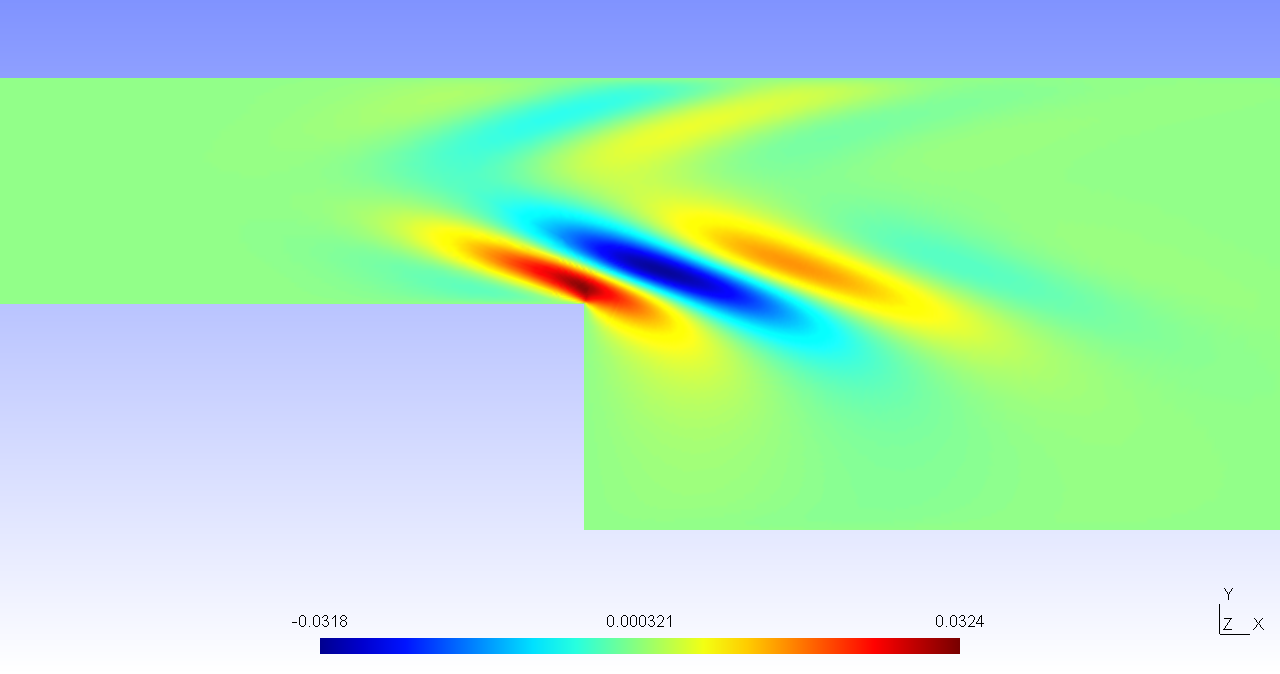Figure 11.22: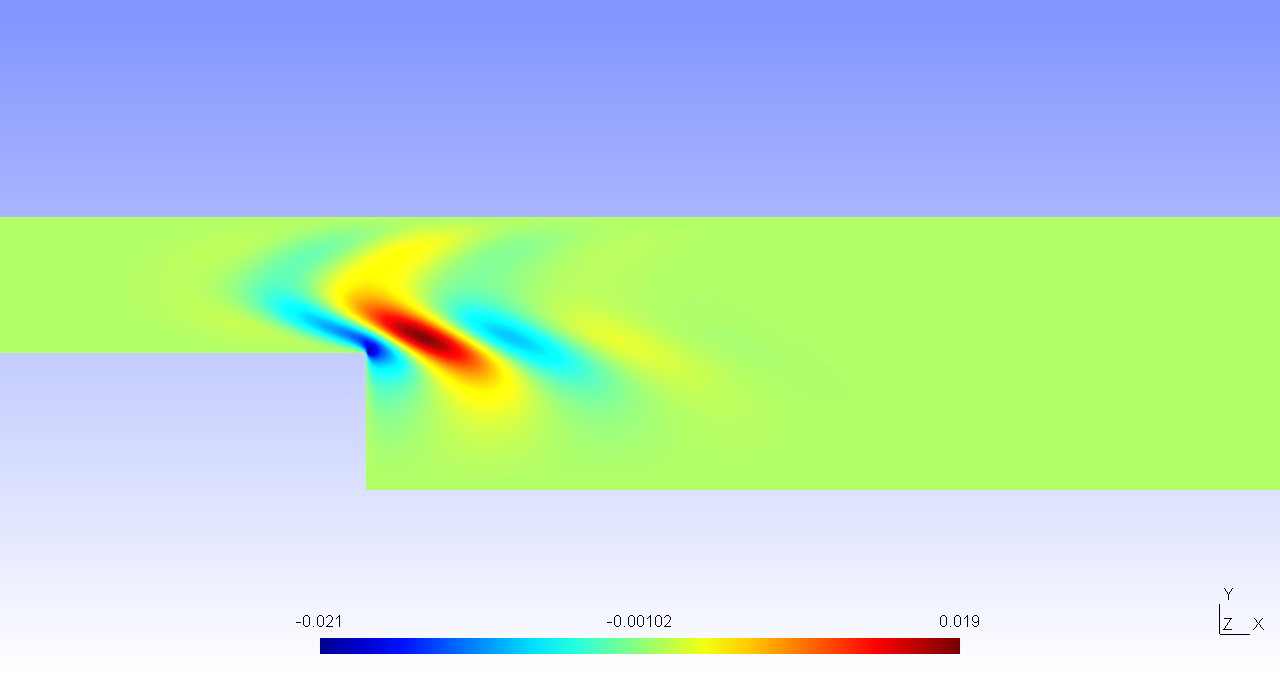Figure 11.23:

It is possible to visualise the transient growth plotting the energy evolution over time if the system is initially perturbed with the leading eigenvector. This analysis was performed for a time horizon τ = 60. It can be seen that the energy grows in time reaching its maximum value at x = 24 and then decays, almost disappearing after 100 temporal units.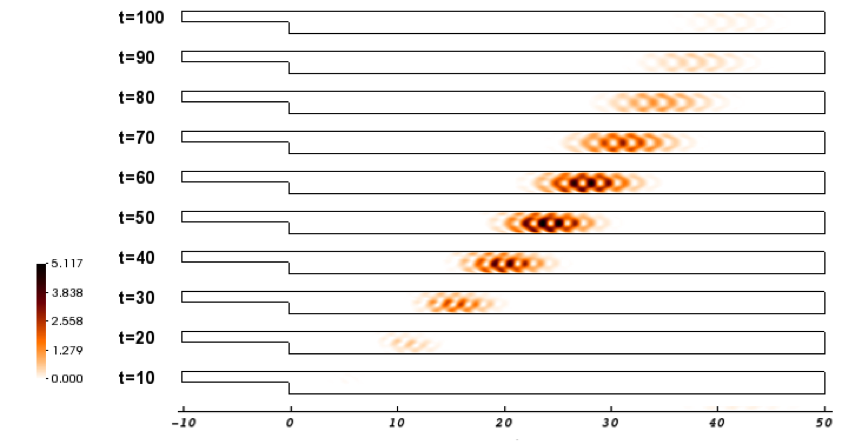Figure 11.24:

#### 11.10.14 BiGlobal Floquet analysis of a of flow past a cylinder

In this example it will be described how to run a BiGlobal stability analysis for a time-periodic base flow using Nektar++. Let us consider a flow past a circular cylinder at Re = 220 has a 2D time-periodic wake that is unstable to a 3D synchronous "mode A" instability.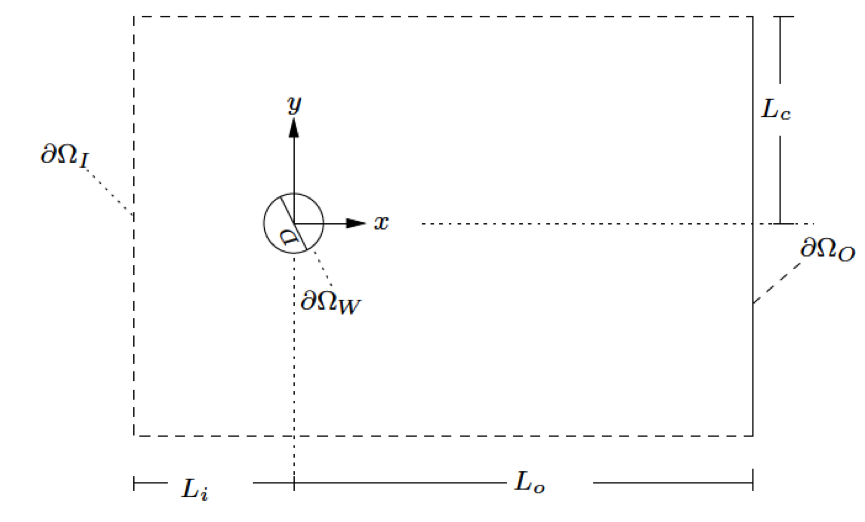Figure 11.25:

##### 11.10.14.1 Background

The numerical solution of the fully three- dimensional linear eigenvalue problem is often computationally demanding and may not have significant advantages over performing a direct numerical simulation. Therefore, some simplifications are required; the most radical consist in considering that the base flow depends only on one spatial coordinate, assuming that the other two spatial coordinates are homogenous. While this method offers a good prediction for the instability of boundary layers, it is not able to predict the instability of Hagen-Poiseuille flow in a pipe at all Reynolds numbers. Between a flow that depends upon one and three-spatial directions, it is possible to consider a steady or time-periodic base flow depending upon two spatial directions and impose three-dimensional disturbances that are periodic in the the third homogeneous spatial direction. This approach is known as BiGlobal stability analysis and it represents the extension of the classic linear stability theory; let us consider a base flow U that is function of only two spatial coordinates: mathbfU(x,y,t). The perturbation velocity can u′ can be expressed in a similar form, but with the dependence on the third homogeneous direction incorporated through the Fourier mode: u′ = û′(x,y,t)eiβz, where β = 2π∕L)and L is the length in the homogeneous direction.

##### 11.10.14.2 Input parameters

In this example we use a mesh of 500 quadrilateral elements with a 6th order polynomial expansion. The base flow has been computed using the Nonlinear evolution operator with appropriate boundary conditions. From its profile, it was possible to determine the periodicity of the flow sampling the velocity profile over time. In order to reconstruct the temporal behaviour of the flow, 32 time slices were considered over one period. Using these data it is possible to set up the stability simulation for a specified β, for example β = 1.7. Let us note that while the base flow is 2D, the stability simulation that we are performing is 3D.

##### 11.10.14.3 Expansion

In this example we will use a 6th order polynomial expansion, i.e. P = 7.

1<EXPANSIONS>
2        <E COMPOSITE="C" NUMMODES="7" TYPE="GLL_LAGRANGE_SEM" FIELDS="u,v,w,p" />
3</EXPANSIONS>
4

##### 11.10.14.4 Parameters
1<TIMEINTEGRATIONSCHEME>
2    <METHOD> IMEX </METHOD>
3    <ORDER> 2 </ORDER>
4</TIMEINTEGRATIONSCHEME>
5
6<SOLVERINFO>
7    <I PROPERTY="SolverType"            VALUE="VelocityCorrectionScheme" />
9    <I PROPERTY="EvolutionOperator"     VALUE="Direct"                   />
10    <I PROPERTY="Projection"            VALUE="Galerkin"                 />
11    <I PROPERTY="ModeType"              VALUE="HalfMode"                 />
12    <I PROPERTY="Driver"                VALUE= "ModifiedArnoldi"         />
13    <I PROPERTY="HOMOGENEOUS"           VALUE="1D"                       />
14</SOLVERINFO>
15

##### 11.10.14.5 Functions
1<FUNCTION NAME="BaseFlow">
2      <F VAR="u,v,p" FILE="cyinder_floq" />
3</FUNCTION>
4

##### 11.10.14.6 Usage

IncNavierStokesSolver session.xml

##### 11.10.14.7 Results

The stability simulation takes about 20 cycles to converge and the leading eigenvalue is λ = 1.2670 with a growth rate σ = 4.7694e - 02. The figure below shows the profile of the magnitude of the eigenmode at z = 2.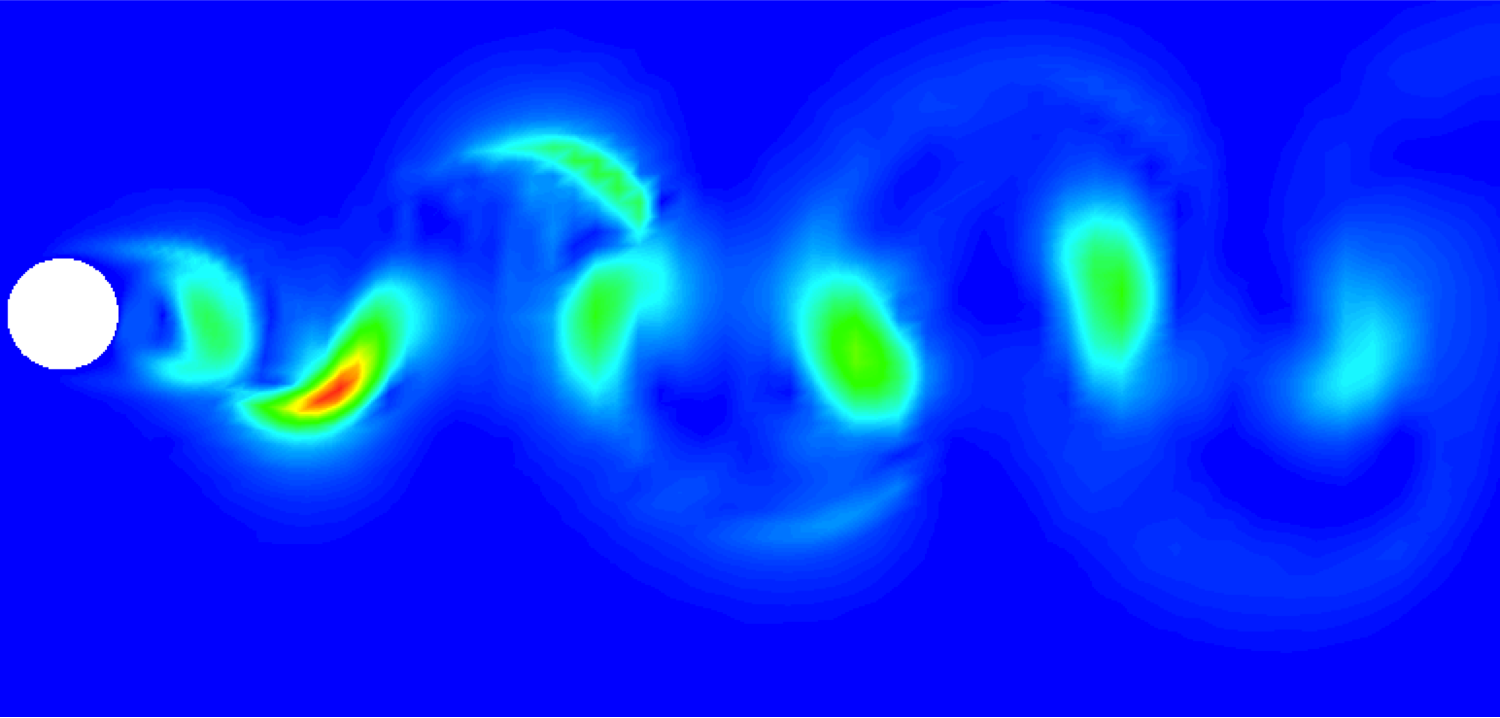Figure 11.26: